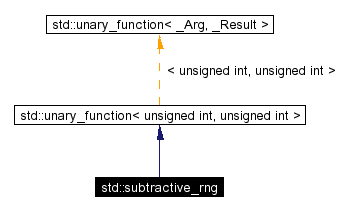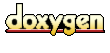Whole document tree

Whole document tree

std::subtractive_rng class Reference
Main Page   Namespace List   Class Hierarchy   Alphabetical List   Compound List   File List   Namespace Members   Compound Members   File Members

# std::subtractive_rng Class Reference

`#include <stl_function.h>`

Inheritance diagram for std::subtractive_rng:[legend]
Collaboration diagram for std::subtractive_rng:[legend]
List of all members.

## Public Methods

unsigned int operator() (unsigned int __limit)
void _M_initialize (unsigned int __seed)
subtractive_rng (unsigned int __seed)
subtractive_rng ()

## Private Attributes

unsigned int _M_table 
size_t _M_index1
size_t _M_index2

## Constructor & Destructor Documentation

 std::subtractive_rng::subtractive_rng ( unsigned int __seed ) ` [inline]`
 Definition at line 534 of file stl_function.h. References _M_initialize().

 std::subtractive_rng::subtractive_rng ( ) ` [inline]`
 Definition at line 535 of file stl_function.h. References _M_initialize().

## Member Function Documentation

 void std::subtractive_rng::_M_initialize ( unsigned int __seed ) ` [inline]`
 Definition at line 515 of file stl_function.h. References _M_index1, _M_index2, and _M_table. Referenced by subtractive_rng().

 unsigned int std::subtractive_rng::operator() ( unsigned int __limit ) ` [inline]`
 Definition at line 508 of file stl_function.h. References _M_index1, _M_index2, and _M_table.

## Member Data Documentation

 size_t std::subtractive_rng::_M_index1` [private]`
 Definition at line 505 of file stl_function.h. Referenced by _M_initialize(), and operator()().

 size_t std::subtractive_rng::_M_index2` [private]`
 Definition at line 506 of file stl_function.h. Referenced by _M_initialize(), and operator()().

 unsigned int std::subtractive_rng::_M_table` [private]`
 Definition at line 504 of file stl_function.h. Referenced by _M_initialize(), and operator()().

The documentation for this class was generated from the following file:
Generated on Mon Apr 8 03:19:35 2002 for libstdc++-v3 Source by1.2.15